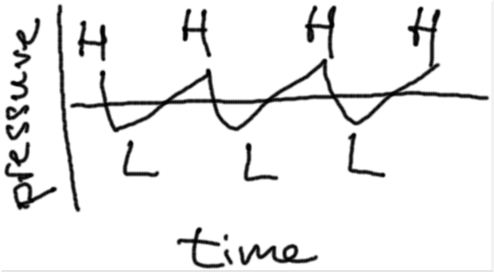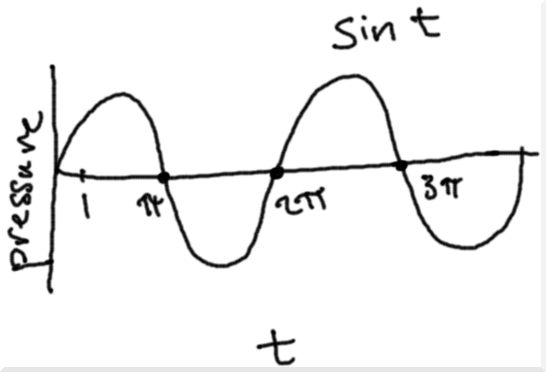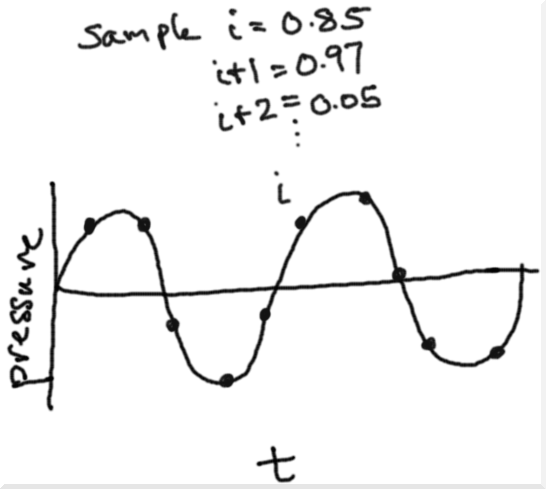# teaching machines

## Generating Digital Audio for a Pitch

Sound is a wave of pressure that travels through the environment. My high school physics teacher Mr. Oppelt told me that pressure is force applied to a surface, and so when we say that music has a beat, we’re quite right. It “beats” on things. Like our ear drums. It beats on other things too, like canyon walls and microphones, and that’s how it gets around.

Sometimes the pressure that sound exerts is higher than what our ear drum is used to. Other times the pressure is lower. A sound wave will oscillate between pressure higher and lower than this equilibrium repeatedly. This leads us to a very simple sketch of a sound wave:A simple model of a sound wave. H means the pressure is higher than the equilibrium. L means the pressure is lower. The pressure oscillates between higher and lower as time progresses.

We want to come up with a mathematical way to represent such a wave. Know any function friend that could help us generate a wave? One that goes above equilibrium and then below equilibrium all day long?

You’re probably thinking sine or cosine. Yeah, those will work. But they aren’t our only choices. However, let’s talk about our other choices another day. For this discussion, we will stick with your sine function. The sine of time t oscillates between -1 and 1 all day long.Our first go at a mathematical model of a sound wave, using the sine function.

The number of oscillations per second determines the sound wave’s pitch. For example, there’s a note, which we call A4, that oscillates 440 times each second. That would make me sick, but A4 is tough.

How can we generate a sound wave for A4, one that oscillates 440 times per second? I’m not just going to dump the equation on you. I want you to think about it. Ask yourself some related questions:

• How many times does plain old sin t oscillate each second?
• How can I alter sin t so that it oscillates once each second?

Regarding the first question, by the time t = 1 second rolls around, sin t hasn’t even started its descent. It doesn’t complete its first oscillation until t = 2 * pi. The proportion of its oscillation completed at t = 1 is 1 / (2 * pi). That’s how many oscillations it completes each second.

Regarding the second question, we want to speed the wave up so that our modified wave does at t = 1 what the original wave did at t = 2 * pi. Well, we can just change our function then to scale t up. When t is 1, make it behave like t = 2 * pi in our original function. Our new expression is sin(2 * pi * t).

What if we want 2 oscillations per second? When t is 1, make it behave like t = 4 * pi = 2 * 2 * pi.

What if we want 3 oscillations per second? When t is 1, make it behave like t = 6 * pi = 3 * 2 * pi.

What if we want F oscillations per second? When t is 1, make it behave like t = F * 2 * pi.

What if we want 440 oscillations per second? Hey, we’re regressing. The general case was just given. Set F = 440.

For any given number of oscillations per second, our model of the pressure wave is sin(F * 2 * pi * t).

With this little formula, if someone gives us a time t and a number of oscillations F, we can tell them how much pressure the wave is applying at that particular moment. That’s interesting, but I want to use this knowledge to generate some digital audio.

Sadly, sound is not typically stored as compact mathematical formulas, probably because most sounds are not pure monotonic pitches and cannot be expressed so simply. Instead of formulas, we create a list or array of discrete samples. Sample i stores the pressure value at the time corresponding to sample i.To digitize a sound wave, we record samples of the pressure at various time values. If we don’t record enough samples, we won’t capture the smoothness of the wave. If we record too many, we waste disk and increase network transfer times.

To adequately capture our sound waves, we usually have to store a lot of samples. I mean it: a lot. A medium quality sampling records 22,050 pressure values each second.

Now, we want to walk along the sound wave and sample the pressure values. First, we make an array large enough to hold all the samples we want:

samplesPerSecond = 22050
nsamples = durationInSeconds * samplesPerSecond
samples = new float[nsamples]

Then we visit each element of the array and drop in its pressure value:

for i in [0, nsamples):
samples[i] = sin(F * 2 * pi * t)

But wait! There’s something wrong with this solution. It won’t even compile. Why?

We’ve never given t, the number of seconds, a value. i and t are kind of similar, but i marks the index of the sample we’re on, not a number of seconds. We need to figure out what sample i’s time value is.

For i = 0, what should t be? 0 seems reasonable.

For i = 1, what should t be? That really depends on how “long” a sample is, or how many samples we’re recording per second. If we’re recording 22,050 samples per second, then each sample is 1 / 22,050 seconds long. In this case, for i = 1, t is 1 / 22050. For i = 2, is 2 / 22050.

In general, t = i / samplesPerSecond.

for i in [0, nsamples):
samples[i] = sin(F * 2 * pi * i / samplesPerSecond)

We now have a collection of digital audio samples for a particular pitch. Whew.

From here, we could head a number of directions: write the array out to a file and play it, figure out how to generate different values of F for notes besides A4, try different oscillating functions, combine and concatenate sample arrays to produce melodies, and so on.